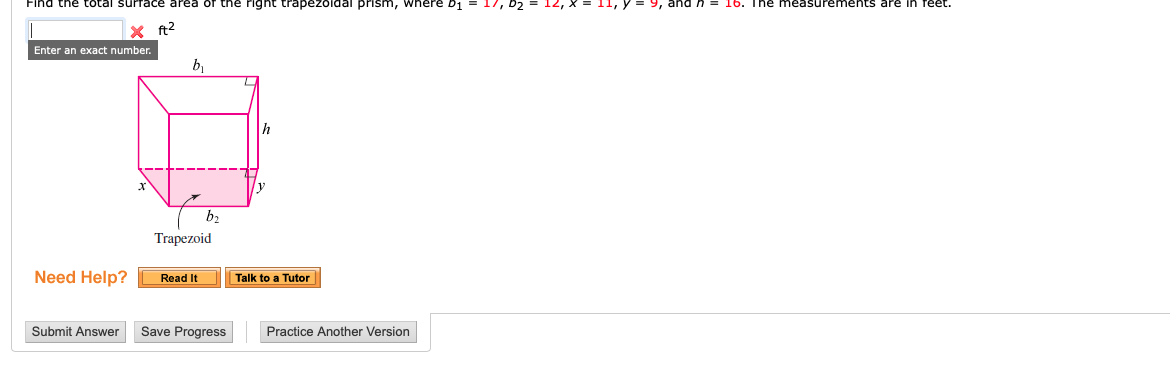# Find the total Surrace area or the right trapezo dal prism, where D1 = 17, D2 = 12, X = 11, yーす, and n = 16· The measurements arereet.Enter an exact numberb2TrapezoidNeed Help? Read ItTalk to a Tutorave Progress Practice Another Version

Question
68 views

Find the total surface area of the right trapezoidal prism, where b1 = 17, b2 = 12, x = 11, y = 9, and h = 16. The measurements are in feet.help_outlineImage TranscriptioncloseFind the total Surrace area or the right trapezo dal prism, where D1 = 17, D2 = 12, X = 11, yーす, and n = 16· The measurements are reet. Enter an exact number b2 Trapezoid Need Help? Read It Talk to a Tutor ave Progress Practice Another Version fullscreen
check_circle

Step 1

The given measures are b1 = 17, b2 = 12, x = 11, y = 9, and h = 16.

Total surface area of the right trapezoidal prism is sum of the 2 times of area of the trapezoidal bases and lateral surface area of the trapezoid.

Step 2

Obtain 2 times the area of the trapezoidal bases.

Step 3

Obtain lateral surface are...

### Want to see the full answer?

See Solution

#### Want to see this answer and more?

Solutions are written by subject experts who are available 24/7. Questions are typically answered within 1 hour.*

See Solution
*Response times may vary by subject and question.
Tagged in

### Geometry